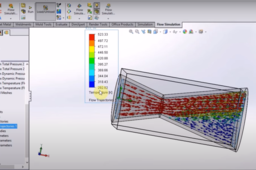# fluid simulation in solidworks for beginnersfluid simulation for begineers (topic covers list down below) :https://youtu.be/bKXdEMGxWPE

1. how to define boundary conditions

2. how to calculate pressure difference

3.how to calculate velocity at a point

4.how to use equation in solidworks flow simulation to calculate desire value

5.how to plot graph in flow animation

6.how to animate flow animation

7.details step for beginners

8.How to set temperature at initial condition